# Selina Solutions Concise Mathematics Class 6 Chapter 19: Fundamental Operations Exercise 19(D)

Selina Solutions Concise Mathematics Class 6 Chapter 19 Fundamental Operations Exercise 19(D) contains answers designed by expert faculty, in accordance with the ICSE exam. The solutions are in a stepwise manner, for better understanding of the concepts among students. Subject matter experts prepare 100% accurate solutions, with the aim to improve the academic performance of students. These solutions also enable them to increase their analytical and logical thinking. Selina Solutions Concise Mathematics Class 6 Chapter 19 Fundamental Operations Exercise 19(D), free PDF links are given below.

## Selina Solutions Concise Mathematics Class 6 Chapter 19: Fundamental Operations Exercise 19(D) Download PDF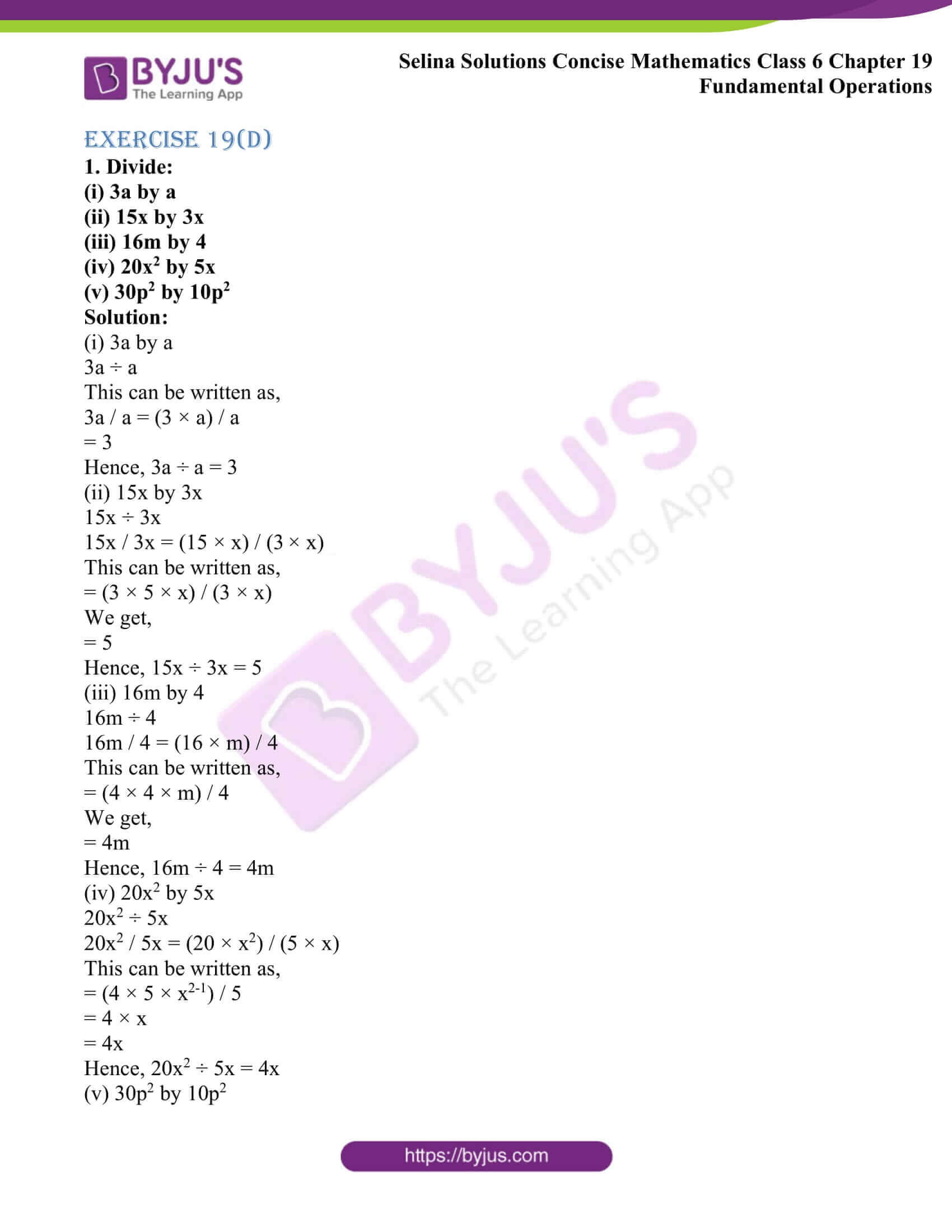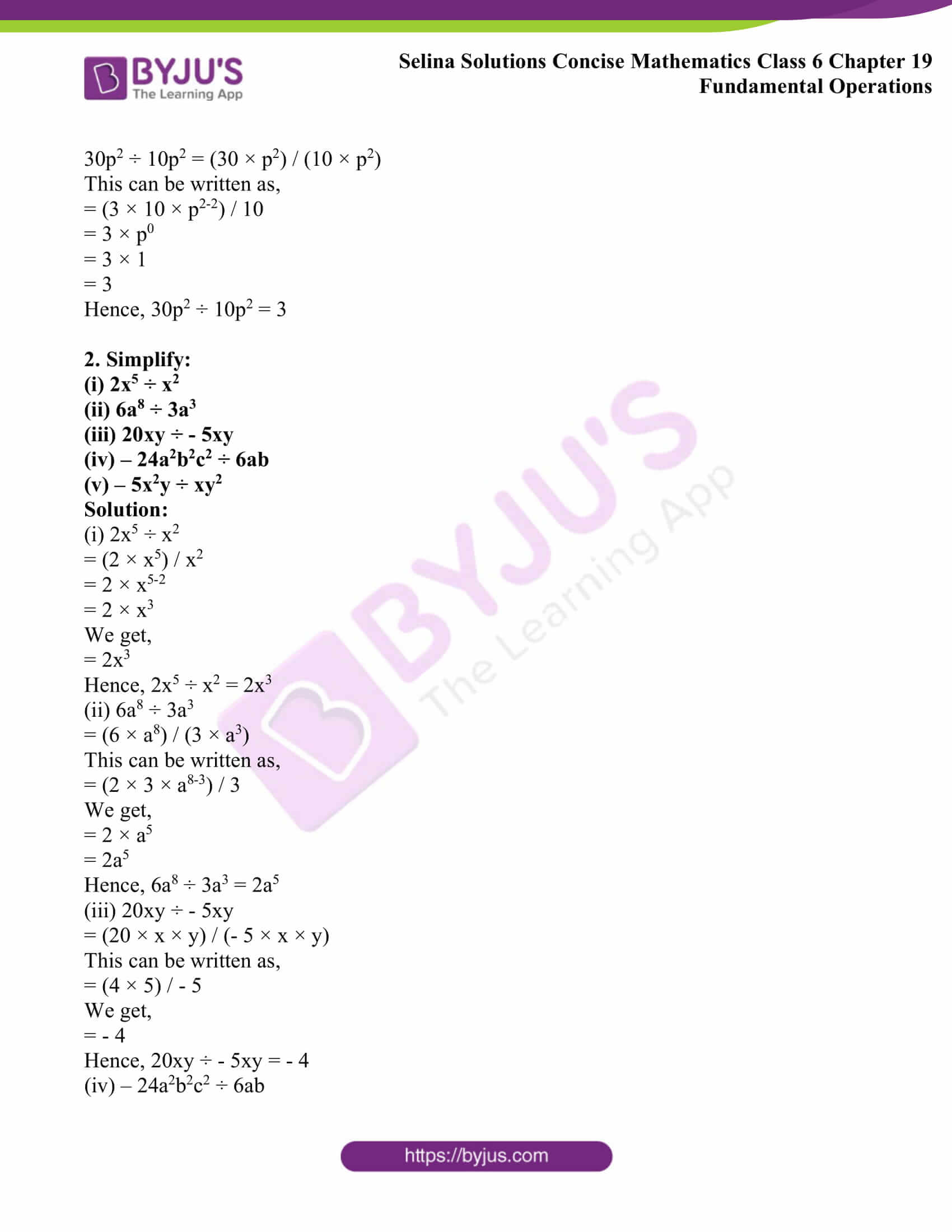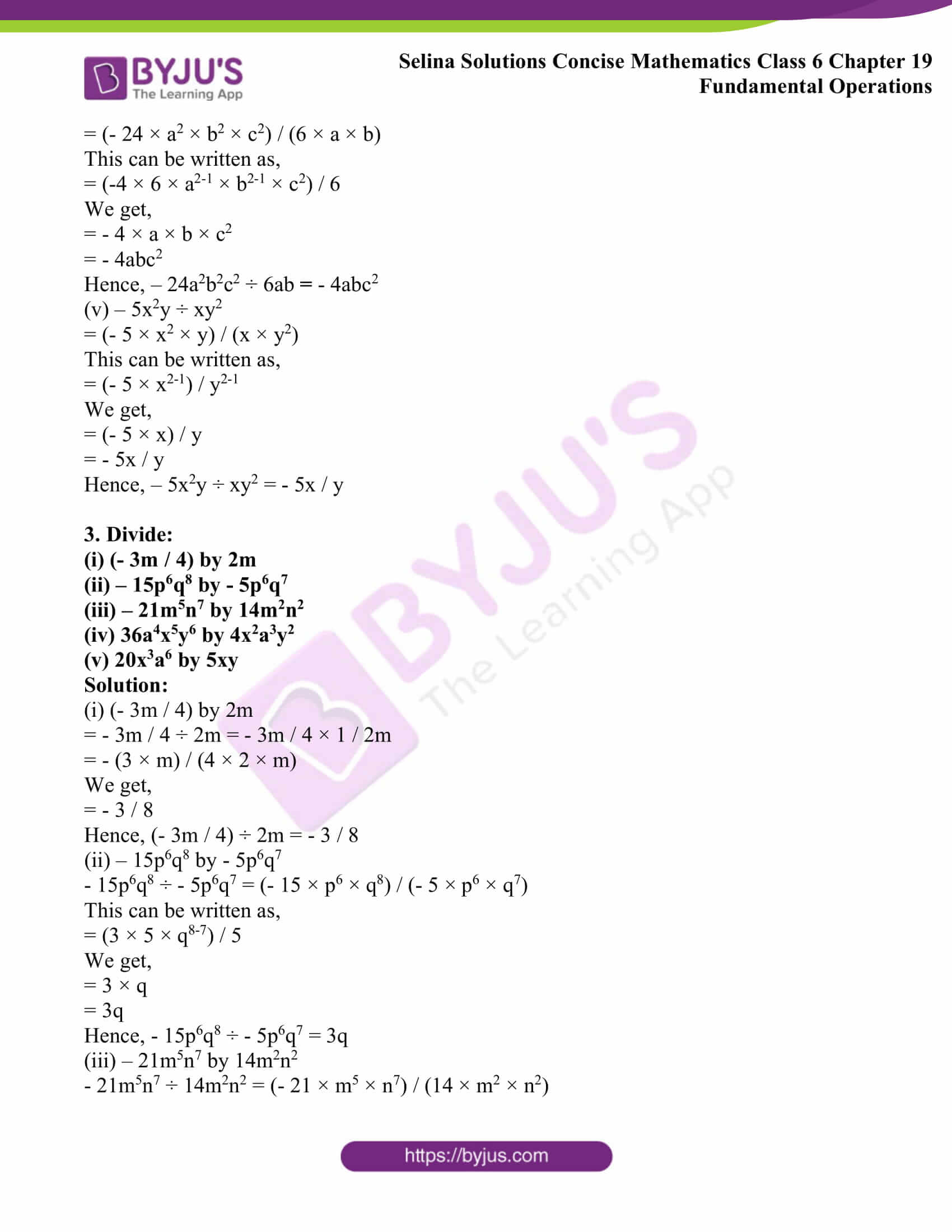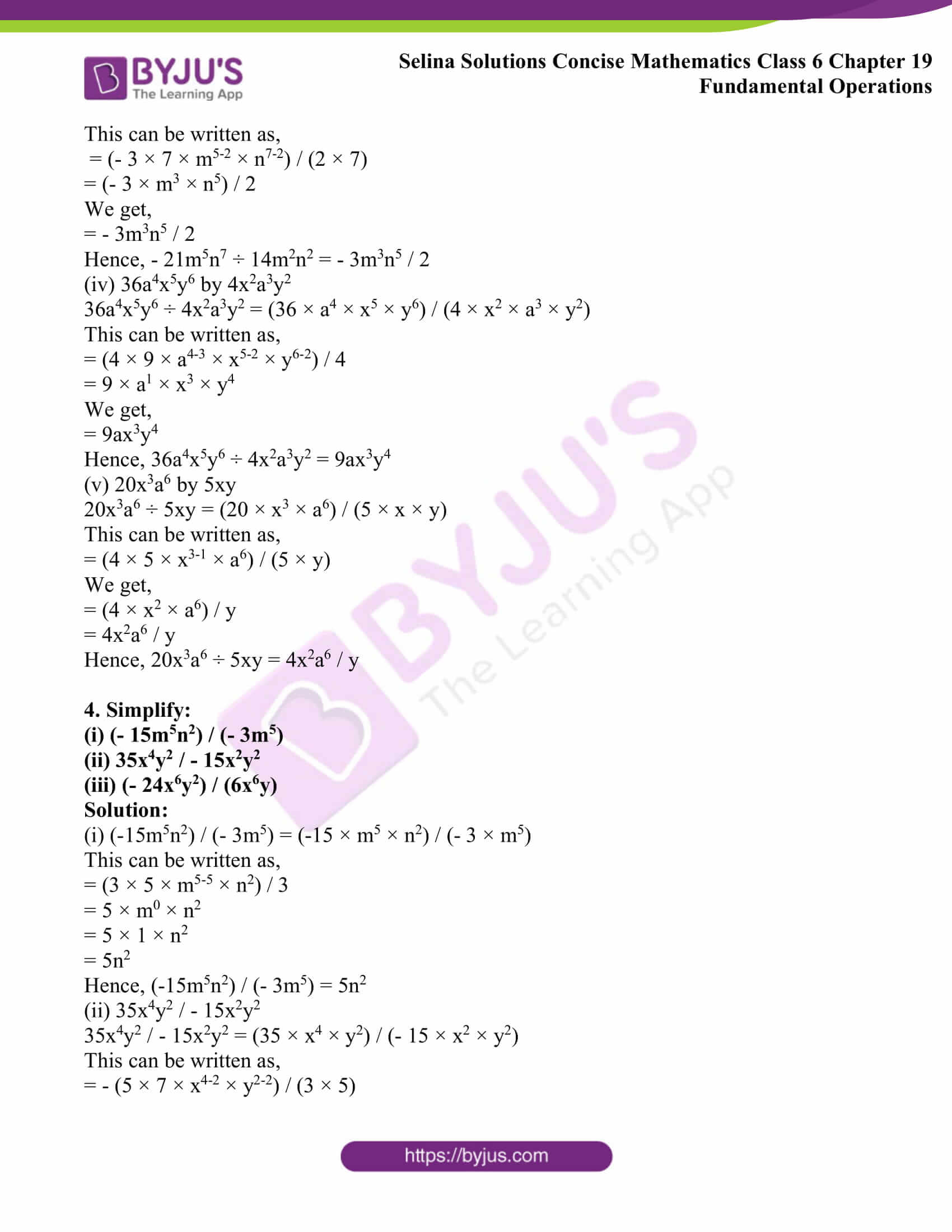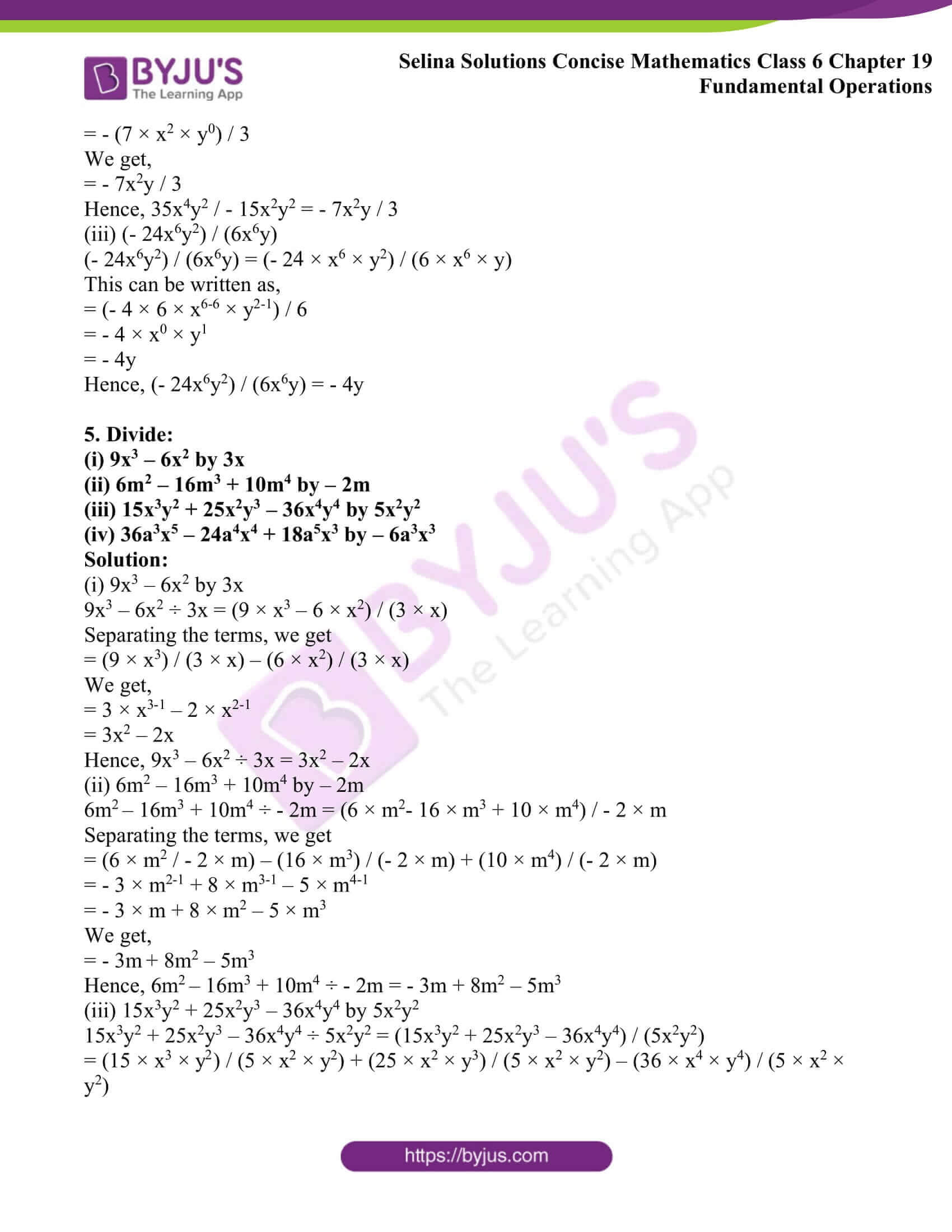### Access other exercises of Selina Solutions Concise Mathematics Class 6 Chapter 19: Fundamental Operations

Exercise 19(A) Solutions

Exercise 19(B) Solutions

Exercise 19(C) Solutions

### Access Selina Solutions Concise Mathematics Class 6 Chapter 19: Fundamental Operations Exercise 19(D)

Exercise 19(D)

1. Divide:

(i) 3a by a

(ii) 15x by 3x

(iii) 16m by 4

(iv) 20x2 by 5x

(v) 30p2 by 10p2

Solution:

(i) 3a by a

3a ÷ a

This can be written as,

3a / a = (3 × a) / a

= 3

Hence, 3a ÷ a = 3

(ii) 15x by 3x

15x ÷ 3x

15x / 3x = (15 × x) / (3 × x)

This can be written as,

= (3 × 5 × x) / (3 × x)

We get,

= 5

Hence, 15x ÷ 3x = 5

(iii) 16m by 4

16m ÷ 4

16m / 4 = (16 × m) / 4

This can be written as,

= (4 × 4 × m) / 4

We get,

= 4m

Hence, 16m ÷ 4 = 4m

(iv) 20x2 by 5x

20x2 ÷ 5x

20x2 / 5x = (20 × x2) / (5 × x)

This can be written as,

= (4 × 5 × x2-1) / 5

= 4 × x

= 4x

Hence, 20x2 ÷ 5x = 4x

(v) 30p2 by 10p2

30p2 ÷ 10p2 = (30 × p2) / (10 × p2)

This can be written as,

= (3 × 10 × p2-2) / 10

= 3 × p0

= 3 × 1

= 3

Hence, 30p2 ÷ 10p2 = 3

2. Simplify:

(i) 2x5 ÷ x2

(ii) 6a8 ÷ 3a3

(iii) 20xy ÷ – 5xy

(iv) – 24a2b2c2 ÷ 6ab

(v) – 5x2y ÷ xy2

Solution:

(i) 2x5 ÷ x2

= (2 × x5) / x2

= 2 × x5-2

= 2 × x3

We get,

= 2x3

Hence, 2x5 ÷ x2 = 2x3

(ii) 6a8 ÷ 3a3

= (6 × a8) / (3 × a3)

This can be written as,

= (2 × 3 × a8-3) / 3

We get,

= 2 × a5

= 2a5

Hence, 6a8 ÷ 3a3 = 2a5

(iii) 20xy ÷ – 5xy

= (20 × x × y) / (- 5 × x × y)

This can be written as,

= (4 × 5) / – 5

We get,

= – 4

Hence, 20xy ÷ – 5xy = – 4

(iv) – 24a2b2c2 ÷ 6ab

= (- 24 × a2 × b2 × c2) / (6 × a × b)

This can be written as,

= (-4 × 6 × a2-1 × b2-1 × c2) / 6

We get,

= – 4 × a × b × c2

= – 4abc2

Hence, – 24a2b2c2 ÷ 6ab = – 4abc2

(v) – 5x2y ÷ xy2

= (- 5 × x2 × y) / (x × y2)

This can be written as,

= (- 5 × x2-1) / y2-1

We get,

= (- 5 × x) / y

= – 5x / y

Hence, – 5x2y ÷ xy2 = – 5x / y

3. Divide:

(i) (- 3m / 4) by 2m

(ii) – 15p6q8 by – 5p6q7

(iii) – 21m5n7 by 14m2n2

(iv) 36a4x5y6 by 4x2a3y2

(v) 20x3a6 by 5xy

Solution:

(i) (- 3m / 4) by 2m

= – 3m / 4 ÷ 2m = – 3m / 4 × 1 / 2m

= – (3 × m) / (4 × 2 × m)

We get,

= – 3 / 8

Hence, (- 3m / 4) ÷ 2m = – 3 / 8

(ii) – 15p6q8 by – 5p6q7

– 15p6q8 ÷ – 5p6q7 = (- 15 × p6 × q8) / (- 5 × p6 × q7)

This can be written as,

= (3 × 5 × q8-7) / 5

We get,

= 3 × q

= 3q

Hence, – 15p6q8 ÷ – 5p6q7 = 3q

(iii) – 21m5n7 by 14m2n2

– 21m5n7 ÷ 14m2n2 = (- 21 × m5 × n7) / (14 × m2 × n2)

This can be written as,

= (- 3 × 7 × m5-2 × n7-2) / (2 × 7)

= (- 3 × m3 × n5) / 2

We get,

= – 3m3n5 / 2

Hence, – 21m5n7 ÷ 14m2n2 = – 3m3n5 / 2

(iv) 36a4x5y6 by 4x2a3y2

36a4x5y6 ÷ 4x2a3y2 = (36 × a4 × x5 × y6) / (4 × x2 × a3 × y2)

This can be written as,

= (4 × 9 × a4-3 × x5-2 × y6-2) / 4

= 9 × a1 × x3 × y4

We get,

= 9ax3y4

Hence, 36a4x5y6 ÷ 4x2a3y2 = 9ax3y4

(v) 20x3a6 by 5xy

20x3a6 ÷ 5xy = (20 × x3 × a6) / (5 × x × y)

This can be written as,

= (4 × 5 × x3-1 × a6) / (5 × y)

We get,

= (4 × x2 × a6) / y

= 4x2a6 / y

Hence, 20x3a6 ÷ 5xy = 4x2a6 / y

4. Simplify:

(i) (- 15m5n2) / (- 3m5)

(ii) 35x4y2 / – 15x2y2

(iii) (- 24x6y2) / (6x6y)

Solution:

(i) (-15m5n2) / (- 3m5) = (-15 × m5 × n2) / (- 3 × m5)

This can be written as,

= (3 × 5 × m5-5 × n2) / 3

= 5 × m0 × n2

= 5 × 1 × n2

= 5n2

Hence, (-15m5n2) / (- 3m5) = 5n2

(ii) 35x4y2 / – 15x2y2

35x4y2 / – 15x2y2 = (35 × x4 × y2) / (- 15 × x2 × y2)

This can be written as,

= – (5 × 7 × x4-2 × y2-2) / (3 × 5)

= – (7 × x2 × y0) / 3

We get,

= – 7x2y / 3

Hence, 35x4y2 / – 15x2y2 = – 7x2y / 3

(iii) (- 24x6y2) / (6x6y)

(- 24x6y2) / (6x6y) = (- 24 × x6 × y2) / (6 × x6 × y)

This can be written as,

= (- 4 × 6 × x6-6 × y2-1) / 6

= – 4 × x0 × y1

= – 4y

Hence, (- 24x6y2) / (6x6y) = – 4y

5. Divide:

(i) 9x3 – 6x2 by 3x

(ii) 6m2 – 16m3 + 10m4 by – 2m

(iii) 15x3y2 + 25x2y3 – 36x4y4 by 5x2y2

(iv) 36a3x5 – 24a4x4 + 18a5x3 by – 6a3x3

Solution:

(i) 9x3 – 6x2 by 3x

9x3 – 6x2 ÷ 3x = (9 × x3 – 6 × x2) / (3 × x)

Separating the terms, we get

= (9 × x3) / (3 × x) – (6 × x2) / (3 × x)

We get,

= 3 × x3-1 – 2 × x2-1

= 3x2 – 2x

Hence, 9x3 – 6x2 ÷ 3x = 3x2 – 2x

(ii) 6m2 – 16m3 + 10m4 by – 2m

6m2 – 16m3 + 10m4 ÷ – 2m = (6 × m2– 16 × m3 + 10 × m4) / – 2 × m

Separating the terms, we get

= (6 × m2 / – 2 × m) – (16 × m3) / (- 2 × m) + (10 × m4) / (- 2 × m)

= – 3 × m2-1 + 8 × m3-1 – 5 × m4-1

= – 3 × m + 8 × m2 – 5 × m3

We get,

= – 3m + 8m2 – 5m3

Hence, 6m2 – 16m3 + 10m4 ÷ – 2m = – 3m + 8m2 – 5m3

(iii) 15x3y2 + 25x2y3 – 36x4y4 by 5x2y2

15x3y2 + 25x2y3 – 36x4y4 ÷ 5x2y2 = (15x3y2 + 25x2y3 – 36x4y4) / (5x2y2)

= (15 × x3 × y2) / (5 × x2 × y2) + (25 × x2 × y3) / (5 × x2 × y2) – (36 × x4 × y4) / (5 × x2 × y2)

On further calculation, we get

= 3 × x3-2 × y2-2 + 5 × x2-2 × y3-2 – (36 × x4-2 × y4-2) / 5

We get,

= 3 × x1 × y0 + 5 × x0 × y1 – (36 × x2 × y2) / 5

= 3x + 5y – (36x2y2) / 5

Hence, 15x3y2 + 25x2y3 – 36x4y4 ÷ 5x2y2 = 3x + 5y – (36x2y2) / 5

(iv) 36a3x5 – 24a4x4 + 18a5x3 by – 6a3x3

36a3x5 – 24a4x4 + 18a5x3 ÷ (– 6a3x3) = (36a3x5 – 24a4x4 + 18a5x3) / – 6a3x3

= (36.a3.x5) / (-6.a3.x3) – (24.a4.x4) / (-6.a3.x3) + (18.a5.x3) / (-6.a3.x3)

We get,

= – 6.x5-3 + 4.a4-3.x4-3 – 3.a5-3

= – 6x2 + 4ax – 3a2

Hence, 36a3x5 – 24a4x4 + 18a5x3 ÷ (– 6a3x3) = – 6x2 + 4ax – 3a2